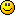#### Topic: Donchian Channel Cross

This indicator uses two donchian channels to give signals , it works fine Shift 2 exceeds Shift1, Shift2 have to be <shift1 for the indicator to work!!!!

Example of working code without Error Msg
IndParam.NumParam.Caption = "Shift 1";
IndParam.NumParam.Value = 1;
IndParam.NumParam.Min = 1;
IndParam.NumParam.Max = 3;

IndParam.NumParam.Caption = "Shift 2";
IndParam.NumParam.Value = 0;
IndParam.NumParam.Min = 0;
IndParam.NumParam.Max = 1;

I spent the whole day trying to code it but cannot find where is the bug to make it work perfect

wishing you all to find the idea useful and waiting for someone to help fix it#### Re: Donchian Channel Cross

What is the error?

#### Re: Donchian Channel Cross

Error: Index was outside the bounds of the array.
Slot type: OpenFilter
Indicator: Donchian Channel Crossover* (High & Low, , , 2, 3)

#### Re: Donchian Channel Cross

FirstBar is incorrect probably, make sure it holds both periods and shifts.

#### Re: Donchian Channel Cross

what do you mean its not holding all periods and shifts !!!  could you elaborate more

i used this :
int   iFirstBar = (iPeriod1 + iShift1 + iPeriod2 + iShift2) + previous + 2;

also i tested all these combinations
//int   iFirstBar = Math.Max(iPeriod1 + iShift1 + previous + 2, iPeriod2 + iShift2 + previous + 2);
//int   iFirstBar = Math.Max(Math.Max(iPeriod1, iShift1), Math.Max(iPeriod2, iShift2)) + previous + 2;
//int   iFirstBar = (int)Math.Max(iPeriod1 + iShift1 + iPeriod2 + iShift2) + 2;
//int   iFirstBar = Math.Max(iPeriod1 + iShift1 + iPeriod2 + iShift2) + previous + 2;
//int   iFirstBar = Math.Max(iPeriod1 + iShift1 + iPeriod2 + iShift2) + 2;
//int   iFirstBar = (iPeriod1 + iShift1 + iPeriod2 + iShift2) + previous + 2;

But all not working !!!!!

#### Re: Donchian Channel Cross

FirstBar is ok then.

Change line 126 to this:

`` for (int iBar = iFirstBar; iBar < Bars-iShift1-iShift2; iBar++)``